# Basic concepts in machine learning

Machine learning algorithms try to imitate the pattern between two datasets in such a way that they can use one dataset to predict the other. Specifically, supervised machine learning is useful for taking what you know as input and quickly transforming it into what you want to know.

In order to explain the basics of supervised learning, I will first start with some basic probability theory. Afterwards, I will describe the “machine learning model”.

# Some probability theory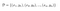Training set consisting of tuples; the y’s are the true labels corresponding to the x’s.

For example, if we wanted to solve an image classification problem we would collect some pictures of dogs, cats and giraffes and then we would have humans go through each of those pictures and label them with their true label. We would use this as training data to learn how to classify new pictures. So, the goal in supervised learning is to learn this function: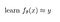We want to learn theta (θ, the parameters) so that this function closely approximates the true label y.

However, there are a few questions we have to answer to actually instantiate a supervised learning method. One important starting point is that predicting probabilities often makes more sense than predicting discrete labels.

1. If we’re allowed to have probabilities, we can much better express what we think is really going on; we might be able to capture the fact that a 4 is likely to be a 4, but it also has a pretty high chance of being a 9. This uncertainty might be useful for making decisions.
2. It’s also easier to learn if we predict probabilities rather than discrete labels, because of smoothness: intuitively we can’t change a discrete label a tiny bit, it’s kind of all or nothing. However, the problem of finding the optimal θ is made easier by the fact that we can change those probabilities by a tiny amount and see if they change in a direction that is closer to what we want.

So, given a training set instead of learning a function that maps x to y, now we’re going to learn a function that maps x to a distribution over y:

Now, we’re going to be taking our input, for example the picture of a dog, and outputting a distribution over its corresponding label y.

Refresher on conditional probabilities

A random variable is defined as a variable whose values depend on outcomes of a random phenomenon. We have two:

• The input x
• The output y

We can write the joint probability of x and y as p(x,y). Joint probability is a statistical measure that calculates the likelihood of two events occurring together and at the same point in time. In other words, joint probability is the probability of event Y occurring at the same time that event X occurs.

Joint probability should not be confused with conditional probability, which is the probability that one event will happen given that another action or event happens.

In fact, joint probability p(x, y) can be decomposed using the chain rule of probability:

The chain rule of probability, not to be confused with the chain rule of calculus, allows the calculation of any member of the joint distribution of a set of random variables using only conditional probabilities.

In fact, you could also rewrite the above equation by dividing both sides by p(x) to get the definition of conditional probability:

So if you know p(x) and p(x, y), you could recover your conditional probability.

We’ll focus on learning p(y|x). In order to do this, we will use the “machine learning method”, which is a three-step program. This way of decomposing learning problems is closely related to Marr’s levels of analysis:

I’ll now list the three steps of the machine learning method with their corresponding Marr’s level.

1 Define your model class: how will you represent your program? This question relates to the middle of the pyramid: the algorithmic level. In other words, what is the structure that is going to carry on our optimization?

2 Define your loss function: how will you measure how good a particular model is? This question relates to the computational level of the pyramid.

3 Pick an optimizer: how will you search through the model class to find the model that minimizes the loss function? This question relates to the implementation level of the pyramid.

The reason that we draw these distinctions is that when we have these different levels of analysis it can help us compartmentalize our thinking and help us to build abstractions so that we can solve the problems in isolation.

We’ll start from the middle of the pyramid, thinking about our representation of the problem.

# 1 How do we represent p(y|x)?

And our goal is to map it to the probability that it is an integer between 0 and 9.

A valid probability distribution consists of positive numbers that sum up to 1.

Let’s look at a simple example:

In the above image, we say that the probability that y is a dog (conditioned on x) is constructed as “x” transpose “θ dog”; the probability that y is a cat (conditioned on x) is constructed as “x” transpose “θ cat”; the parameters are defined by the concatenation of “θ dog” and “θ cat”.

However, this program does not output a valid probability distribution: in general p(y = dog) + p(y = cat) will not sum to 1 unless the θs are chosen very very carefully. Therefore, we’re violating our constraint

Let’s address this problem: instead of having “x” transpose “θ dog” be equal to p(dog), we’re going to define it as equal to some function f dog(x); we thus have some intermediate function inside of our program: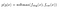Take outputs from f dog and f cat, run them through a softmax

The softmax is going to take in f dog and f cat and it’s going to force those numbers to all be positive and sum up to 1. In theory, it could be any bijective (one-to-one and onto) function, because you want a function that uses the inputs you give.

A function f from the domain to the range is bijective when (1) No element of the range is the image of more than one element in the domain (2) All elements in the range are used.

But apart from this condition, why is it that we can use lots of different functions that make those numbers positive and sum to 1?

The choice of your function in machine learning is not meant to produce the right answer; you produce the right answer by choosing the right parameters. The function just needs to be general enough so that there exists a choice of θ that represents the right answer. For example, an exponential function is a good choice because it maps the entire real number line to the entire positive part of the of the real number line; in a sense, it’s the least restrictive way to turn any number into any positive number

If we make all of our numbers positive by exponentiating them, how do we make that sum to one? With normalization: you take each of the numbers and you divide them by their sum and if you do this then the resulting new numbers will have to sum to 1.

We can now define the softmax function: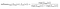It takes all of its inputs. it exponentiates them and then it divides by their sum; in this example, we see the dog output.

In general, you might have n labels (not just a a dog) and p (y|x) is a vector with n elements, one element for every possible label:

You have some function f θ(x), which is a vector-valued function with n outputs that might not be positive and might not sum to one:

So the softmax takes care of this:

Its sole job is to make the numbers positive and to make them sum to 1.

# 2 How do we select our parameters θ?

To start thinking about defining our loss function, let’s start with a with a more basic question: how is our dataset generated? If our dataset consists of pictures and their corresponding labels, the actual process that gave rise to those pictures was probably pretty complex, involving photons and people making decisions about what to photograph and so on.

When it comes to trying to model it, we can come up with a very crude model: we’re going to represent that as a random process; there exists some p(x) distribution over photographs and humans randomly sampling from p(x) when they take photographs.

And the label “dog” is sampled from some distribution over labels, which is not completely random and depends on the photograph:

By the chain of probability, we can compose these two and generate samples (x, y) from their joint distribution p(x,y):

Our dataset is sampled from this distribution and consists of many tuples (x, y), which are generated according to the distribution p (x, y).

To make this a little more formal, what is the probability of seeing a particular training set? We need to make an assumption about how different data points relate to one another. The assumption we’re going to make is that we are dealing with independent and identically distributed data.

• Independent means that every (x, y) tuple is independent of every other (x, y) tuple, meaning that if you observe a cat for x1, this doesn’t change how likely you are to see a cat or a dog for x2
• Identically distributed means that every (xi, yi) comes from the same distribution.

If we have this assumption, we know we can write the probability p(D) of seeing a particular training set as a product over all i of p(xi, yi).

Why? Because “independent” means that the joint p(D) is formed as a product of the marginals p(xi) and p(yi).

In other words, if you flip a coin twice, the probability of seeing heads on the second flip doesn’t depend on the probability of seeing heads on the first flip. So if you want to know the probability of getting heads twice in a row you just multiply the probability of heads on the first attempt by the probability of heads on the second attempt. And because it’s identically distributed that’s the same as just taking the probability of heads and squaring it.

We have a probability associated with our dataset. Since we’re learning p(y|x), we can decompose the above formula using the chain rule of probability:

Now we have a probability associated with our dataset expressed as a product over all of our data points of p(xi) by p(yi|xi). What we are learning is p θ of (y|x). It is important to notice that this is the learned function, which depends on our parameters: this is a model of the true p(y|x). Of course, it’s not the real process by which labels get associated with images, it’s a model of that process. And we’re going to try to get that model to be as accurate as possible

A good model should make the data look probable: one θ is better than another θ if the corresponding p θ(y|x) makes the entire data set look more probable and increases p(D):

However, the issue here is that we express this probability p(D) as a product of a bunch of other probabilities that are between 0 and 1: if you multiply millions of numbers that are between 0 and 1, you’re going to get something very very close to zero. Essentially, this objective is numerically challenging

What we can do instead is we can take the logarithm of p(D). When you take the logarithm of a product, you get a sum of logarithms:

But since p(xi) doesn’t depend on θ, we can just treat is a constant:

We can equivalently say that our objective is a sum over all of our data points of the logarithm of p θ(yi|xi) plus some other stuff that does not depend on θ.

We can formulate the problem of learning θ as our attempt to find the θ that maximizes the log p(D):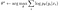This is called maximum likelihood estimation (MLE), because this log probability is the likelihood and we are choosing θ to maximize the likelihood

Often times, we will see this written as a minimization (this is just a convention):This is also called the cross-entropy

We still want to maximize the the log p(D), which is the same as saying that we want to minimize the negative log probability. So, this is our loss function, which quantifies how bad θ is

There are other ways potential way to quantify how bad θ is. For example, you could have a zero-one loss: it just says that you get a a loss of one if you got the wrong answer and you get a loss of zero if you got a right answer:

If you’re doing a continuous regression problem, you might have a mean squared error loss:

If you’re predicting a continuous value number, you might want to be as close to that number as possible.

3 How do we pick our optimizer?

Now we have to do is figure out how to find the setting of θ that minimizes our loss function. One of the things that it helps to think about when imagining optimization algorithms is what’s called the loss landscape. You can think of the loss landscape as a plot of the loss function. L(θ) denotes the loss function.

Let’s say that θ is 2D: you could imagine a plot where the horizontal axes are θ1 and θ2. The vertical axis represents the function L(θ). Just from glancing at it you know where the best θ is: it’s right there at the bottom of that bowl.

We want to basically find the direction in which the loss function decreases and move our θ in that direction. A very general sketch of an algorithm would be:

Find the direction v such that L(θ) decreases and then pick the new θ to be the old θ plus some rate α times v. α is some small constant that is also called the learning rate. Then, we repeat this process enough times and we’ll hopefully settle down at the minimum.

Interestingly, the direction of steepest descent is not actually always the best choice, but what all these methods will have in common is that they will all move in the direction where L(θ) decreases at least locally.

Mathematically speaking, we represent a direction as a vector with n entries that points in the direction where L(θ) will decrease. It is easier to visualize in 1D:

If I’m located at a certain point, the slope of the function at that point tells me in which direction it decreases. If the slope is negative, the function decreases to the right; if the slope is positive, the function decreases to the left:

Just from looking at the slope, we can therefore tell which way to go to decrease the function and the same intuition applies in higher dimensions. In general, for each dimension we should go in the direction opposite to the slope along that dimension.

What is a slope? A slope is just a derivative. All we do is we just set the direction for every dimension to be the negative of the partial derivative of our function L with respect to that dimension of θ.

In 2D, V1 is then:

V2 is:

These are not the only directions in which θ decreases: in fact, there’s an entire half space of vectors that encode directions in which θ decreases. So, these partial derivatives give you the direction of steepest decrease, but if you go a little to the left or to the right, θ will still decrease. Of course, if you go in the opposite direction, θ will increase. As long as you have a positive dot product with v, you will go in a direction where L(θ) decreases. This is to say that it’s not a unique degree structure: it’s the steepest one, which doesn’t necessarily mean it’s the best one.

A very useful concept for doing this is something called the gradient, whose symbol is ∇. The ∇ is a vector; every dimension of that vector is the partial derivative of our function with respect to the corresponding dimension of θ.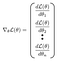The gradient is formed by taking partial derivatives and stacking them up in a vector

Here’s a sketch of the gradient descent algorithm:

1. Compute the gradient step
2. Set θ to be:

We have therefore selected our optimizer

The method we just designed is called logistic regression. Logistic regression is not deep learning, but a lot of the concepts in deep learning take their inspiration from logistic regression.

# Empirical risk minimization

Let’s think back to the zero-one loss, which assigns 0 to loss for a correct classification and 1 for an incorrect classification

You can think of risk as the probability that you will get it wrong. Think back to our generative process: someone takes a picture. This is a randomly sampled picture from the distribution of pictures. It has a label sampled from the true distribution of labels. Given that picture, what’s the probability we’ll get it right or wrong?

The probability you get it wrong is just the expected value of the zero-one loss. Generalizing to other losses, the risk is the expected value of that loss

If we’re using the negative log likelihood, we could say the risk is the expected value under the true distribution over x and the true distribution over y of the loss that we’ll get for that x and y and our learned θ:

The risk is what we want to minimize. However, we can’t calculate true risk, because we can’t get infinite samples. Instead, we’re given a dataset D. So what we can calculate is the empirical risk, which is a sample-based estimate of the true risk. You get this sample-based estimate by averaging your loss over all of your samples.The empirical risk approximates the true risk

Is the empirical risk a good approximation of the true risk? There are two situations where we might have trouble.

1. Overfitting happens when the empirical risk is low, but the true risk is high. This means that your average loss on your training set is low, but but the true expected value of your loss is not low.

Say that I’m trying to fit this line and the dots correspond to my training data: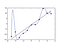Overfitting

A high degree polynomial would perfectly fit the dots and result in very low empirical risk, as it passes right through the points. However, its true risk is going to be quite high. This can happen (1) if the dataset is too small or (2) if the model is too powerful and has too many parameters

2. Underfitting happens when the empirical risk does match the true risk, but they are both high.

For example, the true function here is some kind of curve, but we chose to fit a line to it and the line is not a powerful enough fit to that curve.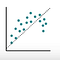Underfitting

This can happen (1) if the model has too few parameters or (2) if your optimizer is not configured well.

# Summary

This function outputs an n dimensional vector for a problem where the label y can take on n different values

For every possible label y, there’s a corresponding θy and each dimension of this vector is given by an inner product between x and the corresponding θy.

You can write this in matrix notation by saying that:

• x is a column vector, which, transposed, results in a row vector
• θ is a row vector; every column of this vector is the θ corresponding to a different label
• so the first column

The probability that y takes on a particular label i is just given by the softmax function applied to the output of fθ(x).

fθ(x) outputs a vector with m entries and the softmax function takes in that vector and outputs the exponential of each entry in that vector divided by the sum of the exponentials of all the entries. This ensures that probabilities are all positive and that they all sum to 1.

We learn θ by using gradient descent and our loss is the negative log likelihood.

Feel free to drop me a message or:

1. Connect and reach me on LinkedIn and Twitter
2. Follow me on Medium

# References

All images taken from Levine, CS182, 2021.

PhD student in Machine Learning — also interested in Neuroscience and Cognitive Science.

## More from Samuele Bolotta

PhD student in Machine Learning — also interested in Neuroscience and Cognitive Science.